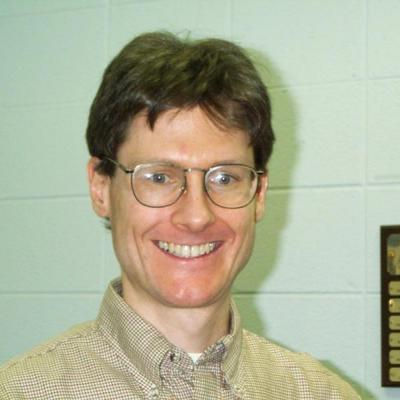# William GrahamProfessor

I am a Professor and the Head of the Department of Mathematics at the University of Georgia. I received my Ph.D. in Mathematics from the Massachusetts Institute of Technology, under the direction of David Vogan.

I have advised three Ph.D. students: Wenjing LiBrandon Samples and Ben Wyser.

Research Areas:
Research Interests:

My research concerns geometry related to algebraic groups. In particular, I am interested in equivariant K-theory, cohomology, and Chow groups, as well as problems related to flag varieties, Schubert calculus, and some related combinatorics.

My work is in the general area of group actions on algebraic varieties. Part of my work concerns the study of general equivariant theories such as equivariant Chow groups and equivariant K-theory. The goals of this work are to prove structure theorems (such as localization theorems) and to describe relations between these theories (such as equivariant Riemann-Roch). A second part of my work concerns the study of specific varieties of interest in Lie theory. These varieties include the flag variety and related objects such as Schubert varieties, nilpotent orbits and Springer fibres. Here I have worked on problems such as positivity in equivariant Schubert calculus, formulas for the restrictions of Schubert classes to fixed points, a new construction of the Belkale-Kumar product on the flag variety, and combinatorics related to rational smoothness of Schubert varieties.

Most of my papers are available in journals or on the arXiv. The journal versions sometimes incorporate changes or corrections that do not appear in the arXiv versions.

Selected Publications:
• Excited Young diagrams, equivariant K-theory and Schubert varieties (with Victor Kreiman). To appear in Transactions of the American Mathematical Society.
• The relative Hochschild-Serre spectral sequence and the Belkale-Kumar product (with Sam Evens). Transactions of the American Mathematical Society 365, 5833-5857.
• The Belkale-Kumar cup product and relative Lie algebra cohomology (with Sam Evens). International Mathematics Research Notices IMRN 2013, no. 8, 1901-1933.
• Components of Springer fibers associated to closed orbits for the symmetric pairs (Sp(n), GL(n)) and (O(n), O(p) x O(q)), II (with L. Barchini and R. Zierau). J. Algebra.
• Smooth components of Springer fibers (with R. Zierau). Ann. Inst. Fourier 61,(2012).
• Multiplication of polynomials on Hermitian symmetric spaces and Littlewood-Richardson coefficients (with Markus Hunziker). Canadian J. Math. (2009).
• On positivity in T-equivariant K-theory of flag varieties (with Shrawan Kumar), Int. Math. Res. Not. IMRN (2008) Volume 2008: article ID rnm 156, 41 pages.
• The forgetful map in equivariant K-theory, Pacific J. Math. 236(2008), 45-55.
• Algebraic cycles and completions of equivariant K-theory (with Dan Edidin), Duke Math. J. 144 (2008), 489-524.
• Nonabelian localization in equivariant $K$-theory and Riemann-Roch for quotients (with Dan Edidin), Adv. Math. 198 (2005), 547-582.
• Nonemptiness of skew-symmetric degeneracy loci, Asian J. Math 9 (2005), 261-271.
• Nonemptiness of symmetric degeneracy loci, Amer. J. Math 127 (2005), 261-292.
• A lookup conjecture for rational smoothness (with Brian Boe), Amer. J. Math. 125 (2003), 317-356.
• Riemann-Roch for quotients and Todd classes of simplicial toric varieties (with Dan Edidin), Comm. Algebra 31 (2003), 3735-3752 (issue in honor of Steven Kleiman).
• Positivity in equivariant Schubert calculus, Duke Math. J. 109 (2001), 599-614.
• Good representations and solvable groups (with Dan Edidin), Michigan Math. J. 48 (2000) (volume for William Fulton's 60th birthday), 203-213.
• Riemann-Roch for equivariant Chow groups (with Dan Edidin), Duke Math. J. 102 (2000), 567-594.
• Equivariant intersection theory (with Dan Edidin), Invent. Math. 131 (1998), 595-634.
• Localization in equivariant intersection theory and the Bott residue formula (with Dan Edidin), Amer. J. Math. 120 (1998), 619-636.
• Algebraic cuts (with Dan Edidin), Proc. Amer. Math. Soc. 126 (1998), 677-685.
• Geometric quantization of nilpotent coadjoint orbits (with David Vogan), in: Geometry and representation theory of real and p-adic groups, Birkhauser, Progress in Mathematics 158,1997.
• The class of the diagonal in flag bundles, J. Diff. Geom. 45 (1997), 471-487.
• Characteristic classes in the Chow ring (with Dan Edidin), J. Alg. Geom. 6 (1997), 431-443.
• Logarithmic convexity of push-forward measures, Invent. Math. 123 (1996), 315-322.
• Characteristic classes and quadric bundles (with Dan Edidin), Duke Math. J. 78 (1995), 277-299.
• Functions on the universal cover of the principal nilpotent orbit, Invent. Math. 108 (1992), 15-27.
Room 302 Boyd Graduate Studies Bldg.

Speaker: William Graham, UGA